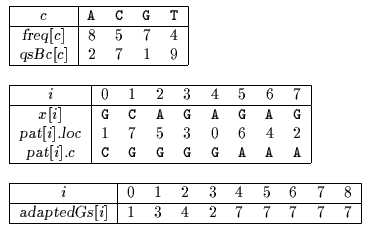Name
• String
• Substring
• Search
Edit

Sunday designed an algorithm where the pattern characters are scanned from the least frequent one to the most frequent one. Doing so one may hope to have a mismatch most of the times and thus to scan the whole text very quickly. One needs to know the frequencies of each of the character of the alphabet.

The preprocessing phase of the Optimal Mismatch algorithm consists in sorting the pattern characters in decreasing order of their frequencies and then in building the Quick Search bad-character shift function (see chapter Quick Search algorithm) and a good-suffix shift function adapted to the scanning order of the pattern characters. It can be done in O(m2+) time and O(m+) space complexity.

The searching phase of the Optimal Mismatch algorithm has a O(mn) time complexity.

Main features:

• variant of the Quick Search algorithm;
• requires the frequencies of the characters;
• preprocessing phase in O(m2+) time and O(m+) space complexity.
• searching phase in O(mn) time complexity.

Example:

Preprocessing phaseTables used by Optimal Mismatch algorithm.

Searching phase

 G C A T C G C A G A G A G T A T A C A G T A C G 1 2 G C A G A G A G

Shift by: 3 (adaptedGs)

 G C A T C G C A G A G A G T A T A C A G T A C G 1 4 3 2 G C A G A G A G

Shift by: 2 (qsBc[A]=adaptedGs)

 G C A T C G C A G A G A G T A T A C A G T A C G 5 1 8 4 7 3 6 2 G C A G A G A G

Shift by: 9 (qsBc[T])

 G C A T C G C A G A G A G T A T A C A G T A C G 1 G C A G A G A G

Shift by: 7 (qsBc[C])

The Optimal Mismatch algorithm performs 15 character comparisons on the example.

C